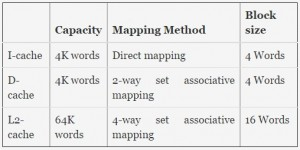Related Articles
GATE | GATE IT 2006 | Question 43
• Last Updated : 19 Nov, 2018

A computer system has a level-1 instruction cache (1-cache), a level-1 data cache (D-cache) and a level-2 cache (L2-cache) with the following specifications:The length of the physical address of a word in the main memory is 30 bits. The capacity of the tag memory in the I-cache, D-cache and L2-cache is, respectively,
(A) 1 K x 18-bit, 1 K x 19-bit, 4 K x 16-bit
(B) 1 K x 16-bit, 1 K x 19-bit, 4 K x 18-bit
(C) 1 K x 16-bit, 512 x 18-bit, 1 K x 16-bit
(D) 1 K x 18-bit, 512 x 18-bit, 1 K x 18-bit

Explanation: Number of blocks in cache = Capacity / Block size = 2m

Bits to represent blocks = m

Number of words in a block = 2n words

Bits to represent a word = n

tag bits = (length of the physical address of a word) – (Bits to represent blocks ) – (Bits to represent a word)

Each block will have it’s own tag bits. So total tag bits = number of blocks x tag bits.

Quiz of this Question

My Personal Notes arrow_drop_up